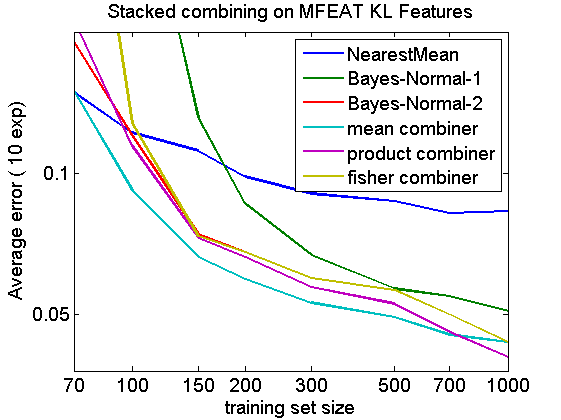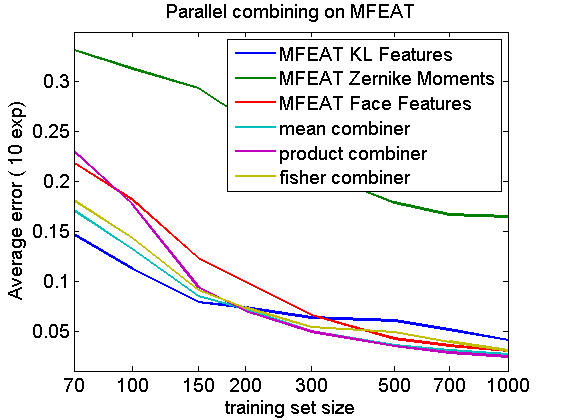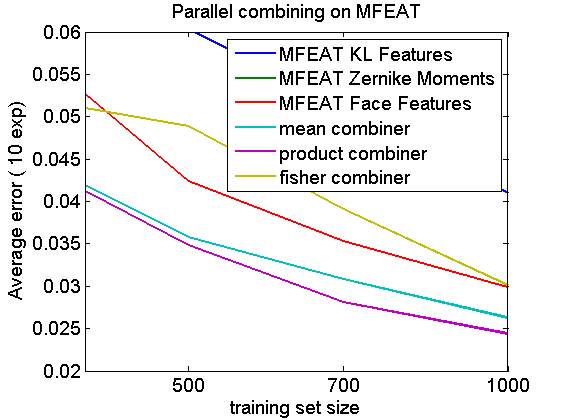# combining classifiers

Introduction of stacked and parallel combining, fixed and trained combiners. It hase to be realized that a combined a set of classifiers can only be better than the best of the constituting base classifiers if this set does not contain an 'optimal' classifier that can solve the problem by its own.

PRTools and PRDataSets should be in the path

Download the m-file from here. See http://37steps.com/prtools for more.

## Contents

```delfigs;
randreset;    % for reproducability
reps = 10;    % repeat experiment reps times.
```

## stacked combining

Three simple classifiers are combined for a 10-class (digits) 64-dimensional problem. Learning curves are computed for the three classifiers and three combiners, two fixed (mean and product) and one trained (fisher).

```r = {nmc ldc qdc([],[],1e-2)}; % three untrained base classifiers
u = [r{:}]*classc; % untrained stacked combiner
a = mfeat_kar;     % the dataset, 10 x 200 objects in 64 dimensions
trainsize = [0.07 0.1 0.15 0.2 0.3 0.5 0.7 0.9999]; % fractions
e = zeros(reps,numel(trainsize),numel(r)+3);
for n=1:reps
[tt,s] = gendat(a,0.5);          % 50-50 split in train and test set
for i=1:numel(trainsize)
randreset(n);                  % takes care that larger training sets
t = gendat(tt,trainsize(i));   % include the smaller ones.
w = t*u;                       % train combiner
for j=1:numel(w.data)          % test base classifiers
e(n,i,j) = s*w.data{j}*testc;
end
e(n,i,j+1) = s*w*meanc*testc;  % test mean combiner
e(n,i,j+2) = s*w*prodc*testc;  % test product combiner
e(n,i,j+3) = s*(t*(w*fisherc))*testc; % train and test fisher combiner
end
end
% plot and anotate
tsizes = ceil(trainsize*size(t,1));
figure; plot(tsizes,squeeze(mean(e)));
set(gca,'xscale','log');
axis([min(tsizes),ceil(max(tsizes)),0.03,0.15]);
set(gca,'xtick',tsizes)
names = char(char(getname(r)),char('mean combiner','product combiner','fisher combiner'));
legend(names);
xlabel('training set size');
ylabel(['Average error ( ' num2str(reps) ' exp)']);
title(['Stacked combining on ' getname(a)])
fontsize(14);
linewidth(2);
```The combiners perform almost everywhere better than the base classifiers. The product rule needs more accurate confidences and thereby a larger training set. The fisher combiner, in fact optimizing a weighted version of the mean combiner, needs more data as well.

## parallel combining

A simple classifier is trained for three aligned feature sets of a 10-class (digits) 64-dimensional problem. Learning curves are computed for the three classifiers and three combiners, two fixed (mean and product) and one trained (fisher).

```a = {mfeat_kar; mfeat_zer; mfeat_fac}; % three feature sets
classf = qdc([],[],1e-2)*classc;       % the classifier, regularized qdc
trainsize = [0.07 0.1 0.15 0.2 0.3 0.5 0.7 0.9999]; % fractions
e = zeros(reps,numel(trainsize),numel(r)+3);
for n=1:reps
[tt,s] = gendat(a,0.5);          % 50-50 split in train and test set
for i=1:numel(trainsize)
randreset(n);                  % takes care that larger training sets
t = gendat(tt,trainsize(i));   % include the smaller ones.
w = t*classf;                  % train the classifier for 3 feature sets
for j=1:numel(a)               % test them
e(n,i,j) = s{j}*w{j}*testc;
end
w = parallel(w);
e(n,i,j+1) = [s{:}]*[w]*meanc*testc;  % test mean combiner
e(n,i,j+2) = [s{:}]*[w]*prodc*testc;  % test product combiner
e(n,i,j+3) = [s{:}]*([t{:}]*(w*fisherc))*testc; % train and test fisher combiner
end
end
% plot and annotate
tsizes = ceil(trainsize*size(t{1},1));
figure; plot(tsizes,squeeze(mean(e)));
set(gca,'xscale','log');
axis([min(tsizes),ceil(max(tsizes)),0.01,0.35]);
set(gca,'xtick',tsizes)
names = char(char(getname(a)),char('mean combiner','product combiner','fisher combiner'));
legend(names);
xlabel('training set size');
ylabel(['Average error ( ' num2str(reps) ' exp)']);
title(['Parallel combining on MFEAT'])
fontsize(14);
linewidth(2);
```The three dataset perform rather differently. For sufficiently large training sets the combiners perform better.

## Show that trained combining is not useful here

```axis([400 1000 0.02 0.06])
```This is the zoomed version of the large training set sizes. It shows that for the trained fisher combiner the training set is still not sufficiently large and does not improve the results of the best feature set.